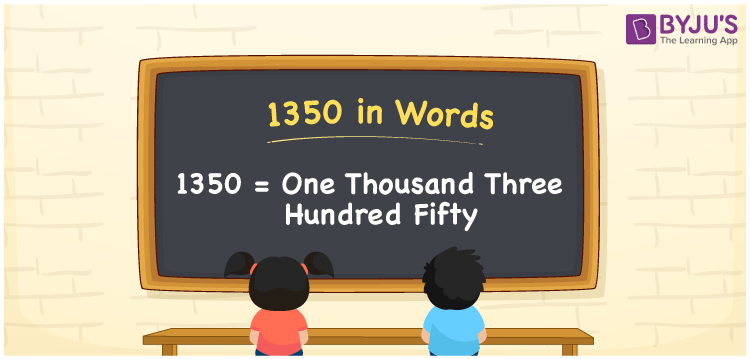# 1350 in Words

We can write 1350 in words as One thousand three hundred fifty. Generally, we use the word form of numbers to express a certain quantity or convey that number to others. Thus, the cardinal number 1350 can be expressed as “One thousand three hundred fifty”. Here in this article, let’s learn how to derive the number 1350 into the word form.

 1350 in words One thousand three hundred fifty One thousand three hundred fifty in Numerals 1350

## 1350 in English Words

Using the English alphabet, we can write numbers in words. So, we can spell 1350 in words as “One thousand three hundred fifty”.## How to Write 1350 in Words?

Let us make the place value chart with four columns since the number 1350 contains four digits, as shown below.

 Thousands Hundreds Tens Ones 1 3 5 0

Here, ones = 0, tens = 5, hundreds = 3, and thousands = 1.

These numbers can be expanded as:

1 × Thousand + 3 × Hundred + 5 × Ten + 0 × One

= 1 × 1000 + 3 × 100 + 5 × 10 + 0 × 1

= 1000 + 300 + 50

= One thousand + three hundred + Fifty

= One thousand three hundred fifty

Hence, 1350 in words = One thousand three hundred fifty.

1350 is a natural number that precedes 1351 and succeeds 1349.

1350 in words – One thousand three hundred fifty

Is 1350 an odd number? – No

Is 1350 an even number? – Yes

Is 1350 a prime number? – No

Is 1350 a composite number? – Yes

Is 1350 a perfect square number? – No

Is 1350 a perfect cube number? – No

## Frequently Asked Questions on 1350 in Words

Q1

### How do you write 1350 in words?

We can write the number 1350 in words as One thousand three hundred fifty.
Q2

### How to write a cheque for 1350 rupees?

For a cheque, we write 1350 rupees in words as One thousand three hundred fifty rupees only.
Q3

### What is the difference between One thousand three hundred fifty and One thousand?

One thousand three hundred fifty – One thousand = 1350 – 1000 = 350 Thus, the difference between One thousand three hundred fifty and one thousand is three hundred fifty.
Test your Knowledge on 1350 in Words